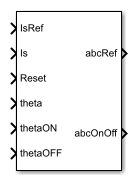# SRM Current Controller

Current control for switched reluctance machines

• Library:
• Simscape / Electrical / Control / SRM Control

•## Description

The SRM Current Controller block performs discrete-time proportional-integral (PI) current control for the Switched Reluctance Machine (SRM) block.

### Equations

To determine the duty cycle, the block implements discrete-time proportional-integral (PI) current control in accordance with this equation.

`$D=\left({K}_{p}+{K}_{i}\frac{{T}_{s}z}{z-1}\right)\left({I}_{s_ref}-{I}_{s}\right)$`

Where:

• D is the duty cycle.

• Kp is the proportional gain.

• Ki is the integral gain.

• Ts is the sample time.

• Is_ref is the reference current.

• Is is the measured current.

To obtain control signals for the three-phases, the block then multiplies the duty cycle with the commutation signals. The resulting three control signals are normalized over the interval [0, 1].

## Ports

### Input

expand all

Reference current for control.

Data Types: `single` | `double`

Measured current.

Data Types: `single` | `double`

External reset signal (rising edge) for the integrator.

Data Types: `Boolean`

Rotor angle in the interval [0, β ].

Data Types: `single` | `double`

Angle for switching on the phase supply.

Data Types: `single` | `double`

Angle for switching on the phase supply.

Data Types: `single` | `double`

### Output

expand all

Control signal normalized in the interval [0, 1].

Data Types: `single` | `double`

Switch signal for the a-, b-, and c-phases. `1` for the switch-on condition and `0` for switch-off condition

Data Types: `single` | `double`

## Parameters

expand all

Proportional gain, Kp, of the controller.

Integral gain, Ki, of the controller.

Anti-windup gain, Kaw, of the controller.

Time, in s, between consecutive block executions. During execution, the block produces outputs and, if appropriate, updates its internal state. For more information, see What Is Sample Time? and Specify Sample Time.

If this block is inside a triggered subsystem, inherit the sample time by setting this parameter to `-1`. If this block is in a continuous variable-step model, specify the sample time explicitly using a positive scalar.

 Saha, N. and S. Panda. "Speed control with torque ripple reduction of switched reluctance motor by Hybrid Many Optimizing Liaison Gravitational Search technique." Engineering Science and Technology. Vol 20 (2017): 909–921.